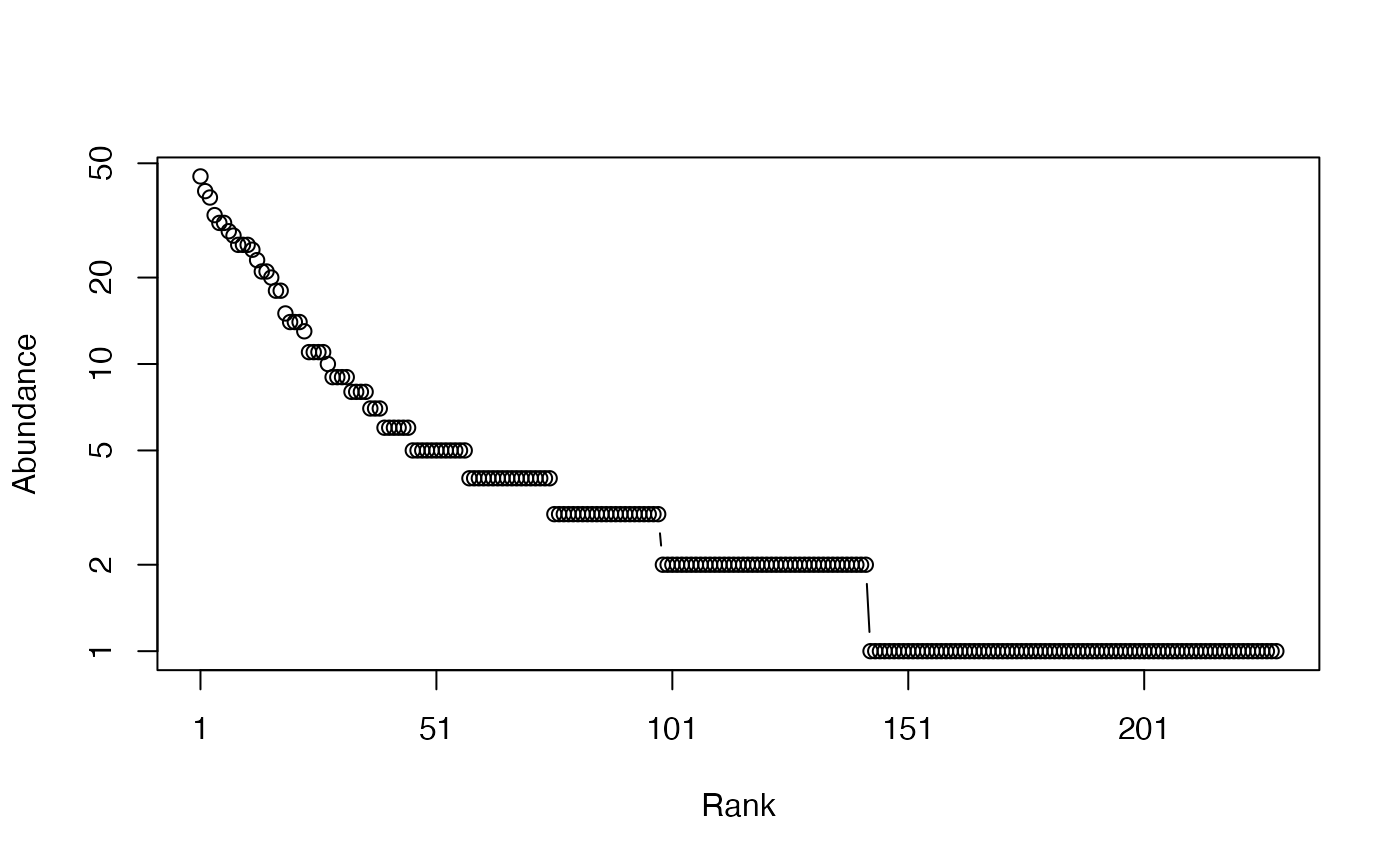Calculates the Simpson entropy of a probability vector.

Simpson(NorP, ...)
bcSimpson(Ns, Correction = "Best", CheckArguments = TRUE)
# S3 method for ProbaVector
Simpson(NorP, ..., CheckArguments = TRUE,
Ps = NULL)
# S3 method for AbdVector
Simpson(NorP, Correction="Best", Level = NULL, ...,
CheckArguments = TRUE, Ns = NULL)
# S3 method for integer
Simpson(NorP, Correction="Best", Level = NULL, ...,
CheckArguments = TRUE, Ns = NULL)
# S3 method for numeric
Simpson(NorP, Correction="Best", Level = NULL, ...,
CheckArguments = TRUE, Ps = NULL, Ns = NULL)

## Arguments

Ps

A probability vector, summing to 1.

Ns

A numeric vector containing species abundances.

NorP

A numeric vector, an integer vector, an abundance vector (AbdVector) or a probability vector (ProbaVector). Contains either abundances or probabilities.

Correction

A string containing one of the possible corrections accepted by bcTsallis or "Lande". "Best", the default value, is currently "Jackknife". Ignored by interpolation and extrapolation.

Level

The level of interpolation or extrapolation. It may be an a chosen sample size (an integer) or a sample coverage (a number between 0 and 1).

...

CheckArguments

Logical; if TRUE, the function arguments are verified. Should be set to FALSE to save time when the arguments have been checked elsewhere.

## Details

Lande's correction has been derived (Lande, 1996; Good, 1953) especially for Simpson entropy, while other corrections are for generalized Tsallis entropy. It is identical to the unbiased estimator proposed by Simpson, although arguments were different. It equals the plug-in etimator multiplied by n/(n-1) where n is the total number of individuals.

Bias correction requires the number of individuals to estimate sample Coverage.

The functions are designed to be used as simply as possible. Simpson is a generic method. If its first argument is an abundance vector, an integer vector or a numeric vector which does not sum to 1, the bias corrected function bcSimpson is called.

Entropy can be estimated at a specified level of interpolation or extrapolation, either a chosen sample size or sample coverage (Chao et al., 2014), rather than its asymptotic value. Simpson's extrapolated entropy estimator does not rely on the estimation of the asymptotic distribution.

## Value

A named number equal to the calculated entropy. The name is that of the bias correction used.

Tsallis, bcSimpson

## Examples

# Load Paracou data (number of trees per species in two 1-ha plot of a tropical forest)
data(Paracou618)
# Ns is the total number of trees per species
Ns <- as.AbdVector(Paracou618.MC\$Ns)
# Whittaker plot
plot(Ns)# Calculate an unbiased estimator of Simpson's index of diversity
Simpson(Ns)
#>    Lande
#> 0.986326